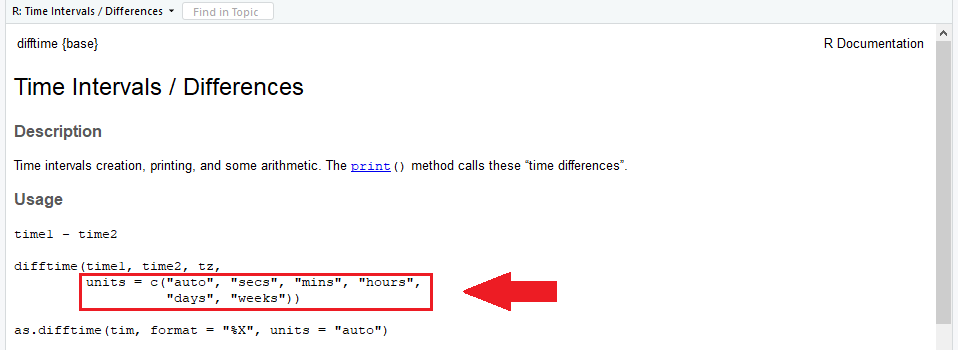# The difftime R Function | 3 Examples (Return Time Difference in Days, Seconds or Weeks)

Basic R Syntax:

`difftime(time_1, time_2)`

The difftime R function calculates the time difference of two date or time objects. The basic syntax for difftime in R is shown above.

In the following article, I’ll show you 3 examples for the usage of difftime in R. Let’s do this!

## Example 1: R difftime – Calculate the Time Difference in Days

As default, the difftime R code calculates the time difference in days. Let’s illustrate that with some example data (i.e. two time characters):

```time_1 <- "2019-08-25 19:09:24" # First time object time_2 <- "2019-08-30 23:09:24" # Second time object```

Now, we can apply the R difftime command to these two time characters:

```difftime(time_1, time_2) # Apply difftime in R # Time difference of -5.166667 days```

The RStudio console returns the sentence: “Time difference of -5.166667 days”. Easy interpretation: there is a difference between our two time characters of 5.166667 days. The minus sign in front implies that the second time character is at a later point in time than the first time character.

## Example 2: R Convert difftime to Seconds

Let’s assume that for our task we need to return the time difference in seconds. No problem for the difftime R function:

```difftime(time_1, time_2, units = "secs") # Apply difftime in seconds # Time difference of -446400 secs```

The time difference of our two dates is 446400 secs. Again, the minus indicates that the second date is after the first date.

## Example 3: Time Difference in Minutes… Hours… Weeks…

Actually, difftime enables the user to calculate the time difference in five different time metrics. Check out the help documentation of the difftime R function:

`?difftime # Check help documentation of difftime`Graphic 1: Screenshot of the difftime Help Documentation in R – Highlighting the available Time Metrics.

As you can see in the highlighted area: difftime provides the time date difference in seconds (as shown in Example 2)…

```difftime(time_1, time_2, units = "secs") # Apply difftime in seconds # Time difference of -446400 secs```

…minutes…

```difftime(time_1, time_2, units = "mins") # Apply difftime in minutes # Time difference of -7440 mins```

…hours…

```difftime(time_1, time_2, units = "hours") # Apply difftime in hours # Time difference of -124 hours```

…days (the default as shown in Example 1)…

```difftime(time_1, time_2, units = "days") # Apply difftime in days # Time difference of -5.166667 days```

…and weeks:

```difftime(time_1, time_2, units = "weeks") # Apply difftime in weeks # Time difference of -0.7380952 weeks```

## Handling Dates – Video Instructions

In general, handling dates in R is a special topic. If you are struggling with the conversion or the handling of date data objects, I can recommend the following video tutorial of the YouTuber Vincent Knight: